An infinite current carrying wire, carrying c...
An infinite current carrying wire, carrying current I is bent in V shape, lying in x-y plane as shown in figure. Intensity of magnetic field at point P will be (take OP = 2a)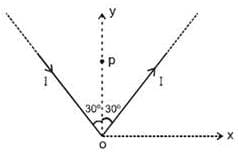• a)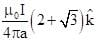• b)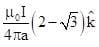• c)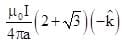• d)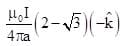Correct answer is option 'A'. Can you explain this answer?

### Related TestRahul Garg Sep 19, 2019Related An infinite current carrying wire, carrying current I is bent in V shape, lying in x-y plane as shown in figure. Intensity of magnetic field at point P will be (take OP = 2a)a)b)c)d)Correct answer is option 'A'. Can you explain this answer?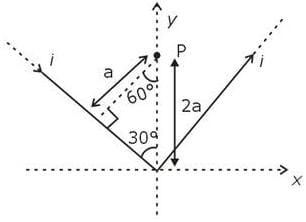B at P due to 1 wire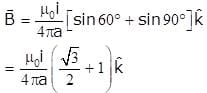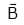due to both wire = 2(is in same direction)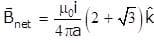1 Crore+ students have signed up on EduRev. Have you?